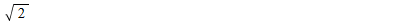## 2233 Reputation

18 years, 13 days

## E8 sphere packing...

Maple

According to Sphere Packing Solved in Higher Dimensions, the best way, i.e., most compact way, to pack spheres in dimensions 8 and 24 are done with the E8 lattice and Leech lattice, respectively. According to the Wikipedia article Leech lattice, the number of spheres that can be packed around any one sphere is 240 and 196,560 (!), respectively, the latter number of spheres counter-intuitively large. It inspired me to try to check that there is indeed room in these lattices for (at least) this number of spheres.

Starting with the E8 lattice: It is generated by the sum (over the integers) of all the 240 roots of E8. Following the prescription given in the subsection 'Construction' in the Wikipedia article E8 (mathematics), these roots may be constructed as follows:

`ROOTS := map(x -> Vector(x),[   # Coordinates all integers: 112 roots   combinat[permute]([+1,+1,0,0,0,0,0,0])[],   combinat[permute]([+1,-1,0,0,0,0,0,0])[],   combinat[permute]([-1,-1,0,0,0,0,0,0])[],   # Coordinates all half-integers: 128 roots   seq(combinat[permute]([      (+1/2)\$(  2*n),   # Even number of +1/2      (-1/2)\$(8-2*n)    # Even number of -1/2   ])[],n = 0..4)]):`

This Maple code gives a list of 240 eight-dimensional vectors. All these roots have the same length (the lattice thus being simply laced):

`convert(map(x -> Norm(x,2),ROOTS),set)[];`If the distance between any pair of different roots is at least this length, then there will be room for 240 spheres of radius equal to this length around any one single sphere. And that is indeed the case:

`DIST_ROOTS := Matrix(nops(ROOTS)\$2,(i,j) ->   Norm(ROOTS[i] - ROOTS[j],2),shape = symmetric):min(convert(DIST_ROOTS,set) minus {0});`Using the above method for the Leech lattice will fail on grounds of hopeless performance, not the least because DIST_ROOTS will take ages to calculate, if at all possible. So any reader is welcome to weigh in with ideas on how to check the Leech lattice case.

PS: By the way, I was surprised to find that the three exceptional Lie algebras E6, E7, and E8 are seemingly not accessible through the Maple command SimpleLieAlgebraData, see its help page. Only the four infinite families A,B,C,D, as well as the two exceptional Lie algebras G2 and F4 are. Using Maple 17, I would like to know if that has been changed in Maple 17+, and if not, why not.

Maple

It seems to me that the page on Unanswered Questions contains questions which are actually already answered.

An example is Sorting a list of xy pairs, on which one I spend some time yesterday preparing an answer, until I discovered that it had already been answered (one of the answers being completely analogous to the one I had prepared).

## Tabbing problem resolved?...

Maple

Has the 'tabbing nuisance', as reported in I declare defeat: rolling back to Maple 9.5, been resolved in Maple 12+? And what about the 'XML-contamination', as also mentioned?

PS: Congratulations to MaplePrimes for a visually very appealing interface. With the migration to this new interface, I have finally been able to gain again access to my account, this partly explaining...

## Maple tag, still ......

Could someone at MapleSoft please tell me how to make the output of something like

```table[table[1,2],table[3,4]];
```

format nicely under the maple-tag? It is, of course, conceivable that it is just me having fundamentally misunderstood the (new?) syntax of the maple-tag. Also, it is conceivable that the Maple tag only malfunctions in the preview version of my posts, but I will never know because as long as it fails there, I will not hit the submit button.

## Attributes...

First, consider the following two analogous codes:

```restart:
x := setattribute({1,2},parity):
x,attributes(x);
```
```restart:
x := setattribute([1,2],parity):
x,attributes(x);
```

Both in Maple 9.5 and Maple 11 they produce the expected output; `{1, 2}, parity` and `[1, 2], parity`, respectively. Next, consider the following two analogous codes:

 1 2 3 4 5 6 7Last Page 1 of 12
﻿